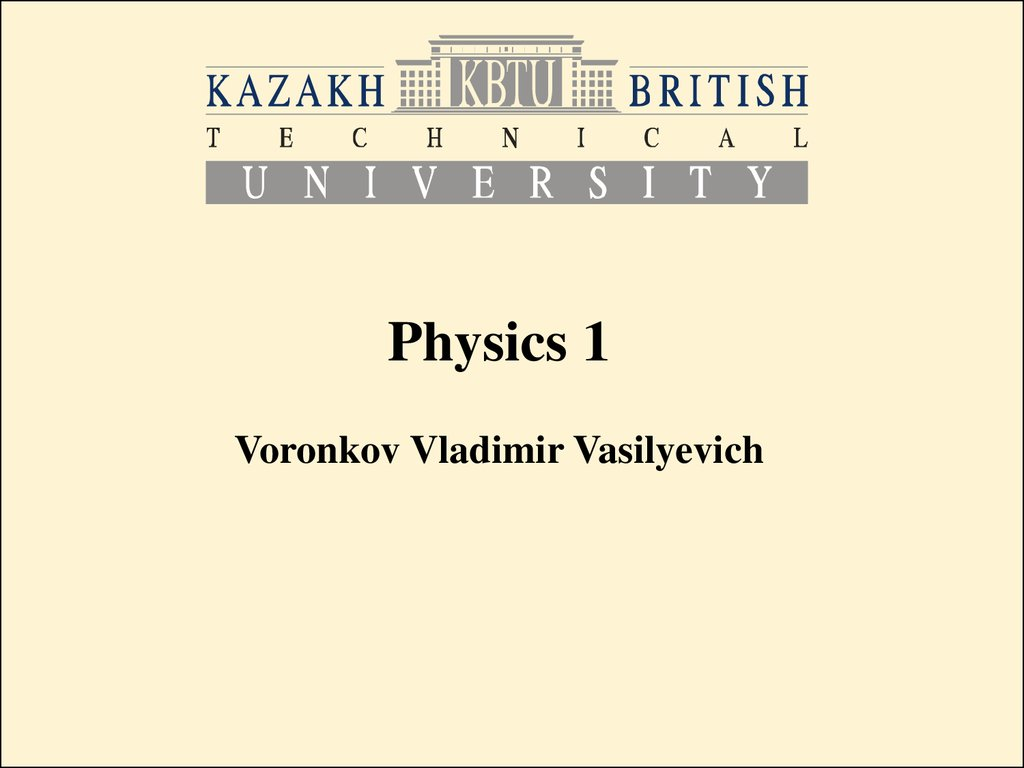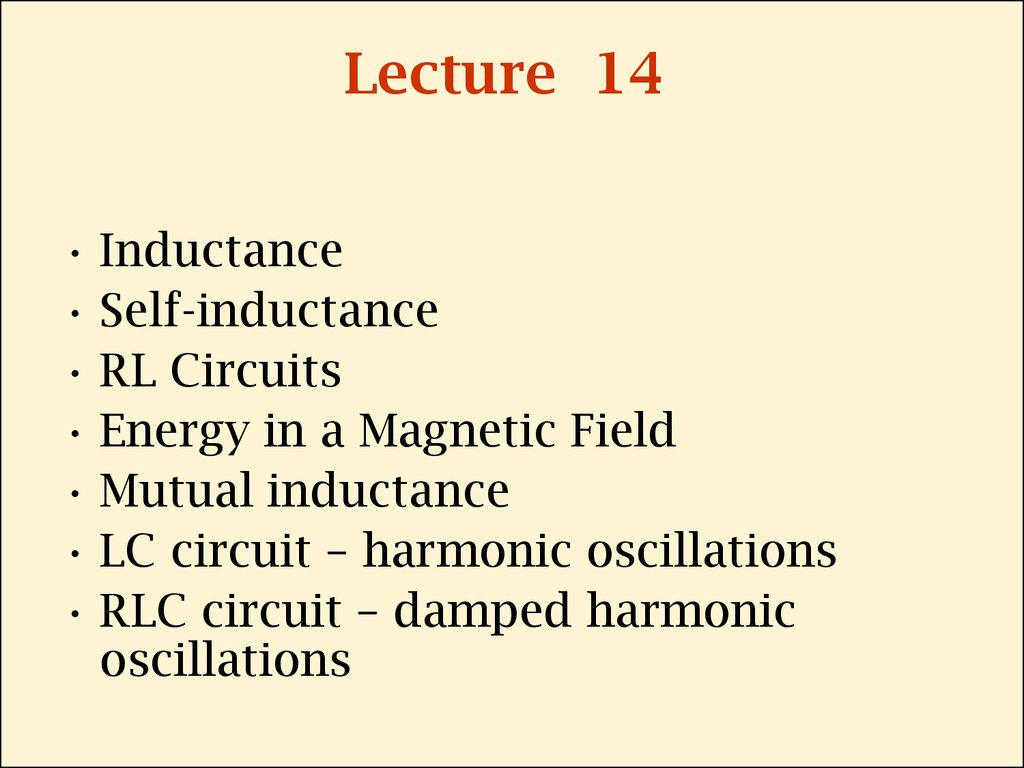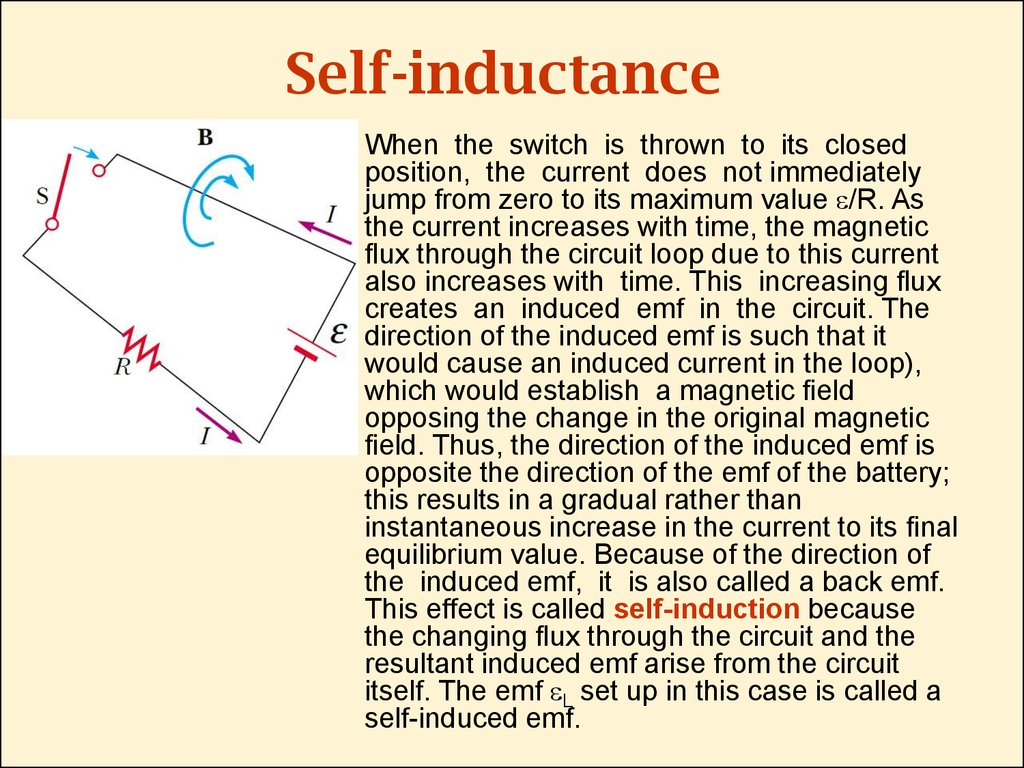# Inductance. Self-inductance

Physics 1

## 2. Lecture 14

• Inductance
• Self-inductance
• RL Circuits
• Energy in a Magnetic Field
• Mutual inductance
• LC circuit – harmonic oscillations
• RLC circuit – damped harmonic
oscillations

## 3. Self-inductance

When the switch is thrown to its closed
position, the current does not immediately
jump from zero to its maximum value e/R. As
the current increases with time, the magnetic
ux through the circuit loop due to this current
also increases with time. This increasing ux
creates an induced emf in the circuit. The
direction of the induced emf is such that it
would cause an induced current in the loop),
which would establish a magnetic eld
opposing the change in the original magnetic
eld. Thus, the direction of the induced emf is
opposite the direction of the emf of the battery;
this results in a gradual rather than
instantaneous increase in the current to its nal
equilibrium value. Because of the direction of
the induced emf, it is also called a back emf.
This effect is called self-induction because
the changing ux through the circuit and the
resultant induced emf arise from the circuit
itself. The emf eL set up in this case is called a
self-induced emf.

## 4.

(a) A current in
the coil
produces a
magnetic eld
directed to the
left.
(b) If the current
increases, the
increasing magnetic
ux creates an
induced emf in the
coil having the
polarity shown by
the dashed battery.
(c) The polarity
of the induced
emf reverses if
the current
decreases.

## 5. Self-induced emf

From Faraday’s law follows that the induced emf is
equal to the negative of the time rate of change of the
magnetic ux. The magnetic ux is proportional to the
magnetic eld due to the current, which in turn is
proportional to the current in the circuit. Therefore,
a self-induced emf is always proportional to the time
rate of change of the current:
L is a proportionality constant—called the inductance
of the coil—that depends on the geometry of the coil
and other physical characteristics.

## 6.

• From last expression it follows that
• So inductance is a measure of the
opposition to a change in current.

## 7. Ideal Solenoid Inductance

Combining the last expression with
Faraday’s law, eL = -N dFB/dt, we see that
the inductance of a closely spaced coil of N
turns (a toroid or an ideal solenoid) carrying
a current I and containing N turns is

## 8. Series RL Circuit

An inductor in a circuit opposes changes in
the current in that circuit:

## 9.

Change of variables: x = (e/R) – I
dx = - dI
where x0 is the value of x at t = 0.

## 10.

• Taking the antilogarithm of the last result:
• Because I = 0 at t = 0, we note from the
de nition of x that x0 = e/R. Hence, this last
expression is equivalent to

## 11.

So the current gradually approaches its
maximum:
The time constant t is
the time interval
required for I to reach
0.632 (1-e-1) of its
maximum value.

## 12. Energy in an Inductor

Multiplying by I the expression for RL–circuit we
obtain:
So here Ie is the power output of the battery, I2R
is the power dissipated on the resistor, then
LIdI/dt is the power delivering to the inductor.
Let’s U denote as the energy stored in the
inductor, then:

## 13.

• After integration of the last formula:
• L is the inductance of the inductor,
• I is the current in the inductor,
• U is the energy stored in the magnetic field
of the inductor.

## 14. Magnetic Field Energy Density

• Inductance for solenoid is:
• The magnetic field of a solenoid is:
• Then:
• Al is the volume of the solenoid, then the
energy density of the magnetic field is:

## 15.

• uB is the energy density of the magnetic field
• B is the magnetic field vector
• m0 is the free space permeability for the
magnetic field, a constant.
• Though this formula was obtained for
solenoid, it’s valid for any region of space
where a magnetic field exists.

## 16. Mutual Inductance

A cross-sectional view of two adjacent
coils. The current I1 in coil 1, which
has N1 turns, creates a magnetic eld.
Some of the magnetic eld lines pass
through coil 2, which has N2 turns.
The magnetic ux caused by the
current in coil 1 and passing
through coil 2 is represented by
F12. The mutual inductance M12 of
coil 2 with respect to coil 1 is:
Mutual inductance depends on the geometry of both circuits
and on their orientation with respect to each other. As the
circuit separation distance increases, the mutual inductance
decreases because the ux linking the circuits decreases.

## 17.

• Mutual inductance depends on the
geometry of both circuits and on their
orientation with respect to each other. As
the circuit separation distance increases,
the mutual inductance decreases because
the ux linking the circuits decreases.

## 18.

• The emf induced by coil 1 in coil 2 is:
• The preceding discussion can be repeated to
show that there is a mutual inductance M21.
The emf induced by coil 1 in coil 2 is:
• In mutual induction, the emf induced
in one coil is always proportional to
the rate at which the current in the
other coil is changing.

## 19.

Although the proportionality constants M12
and M21 have been obtained separately, it
can be shown that they are equal. Thus, with
M12 = M21 = M, the expressions for induced emf
takes the form:
These two expression are similar to that for the
self-induced emf: e = - L(dI/dt).
The unit of mutual inductance is the henry.

## 20. LC Circuit Oscillations

• If the capacitor is initially
charged and the switch
is then closed, we nd
that both the current in
the circuit and the
charge on the capacitor
oscillate between
maximum positive and
negative values.
• We assume:
– the resistance of the circuit is
zero, then no energy is
dissipated,
– energy is not radiated away
from the circuit.

## 21.

• The energy of the LC system is:
• U=const as we supposed no energy loss:
• Using that I=dQ/dt we can write:

## 22.

• The solution for the equation is:
• The angular frequency of the oscillations
depends solely on the inductance and
capacitance of the circuit. This is the
natural frequency (частота собственных
колебаний) of oscillation of the LC circuit.

## 23.

• Then the current is:
• Choosing the initial conditions: at t = 0, I = 0
and Q = Qmax we determine that f=0.
• Eventually, the charge in the capacitor and the
current in the inductor are:

## 24.

Graph of charge versus
time
and
Graph of current versus
time for a resistanceless,
NOTE: Q and I are 90° out
of phase with each other.

## 25.

• Plots of UC versus t
and UL versus t for a
resistanceless,
• The sum of the two
curves is a constant
and equal to the total
energy stored in the
circuit.

A series RLC
circuit. Switch
S1 is closed and
the capacitor is
charged. S1 is
then opened
and, at t = 0,
switch S2 is
closed.

## 27.

• Energy is dissipated on the resistor:
• Using the equation for dU/dt in the LC-circuit
(slide 2):
• Using that I=dQ/dt:

## 28.

• The RLC circuit is analogous to the damped
harmonic oscillator, where R is damping coefficient.
• Here b is damping coefficient. When b=0, we have
pure harmonic oscillations.
• Solution is:
• RC is the critical resistance:
• When R<RC oscillations are damped harmonic.
• When R>RC oscillations are damped unharmonic.

## 29.

• The charge decays in damped harmonic
way when

## 30.

• Charge decays in damped inharmonic way
when R>RC, then the RLC circuit is
overdamped.

## 31. Units in Si

H (henry): 1H=V*s/A
• Mutual Inductance M H (henry): 1H=V*s/A
• Energy density
u J/m3
• Inductance
L